Refer to our Texas Go Math Grade 4 Answer Key Pdf to score good marks in the exams. Test yourself by practicing the problems from Texas Go Math Grade 4 Lesson 5.2 Answer Key Add Fractions Using Models.

Essential Question

How can you add fractions with like denominators using models?
We can directly add the numerators
if there is like denominators using models

Unlock the Problem

Ms. Clark made a loaf of bread. She used $$\frac{1}{8}$$ of the bread for a snack arid $$\frac{5}{8}$$ of the bread for lunch. How much bread did she use for a snack and lunch?

One Way Use a picture.

$$\frac{1}{8}$$ is ___________ eighth-size piece of bread.
$$\frac{1}{8}$$ is ___________ eighth-size pieces of bread.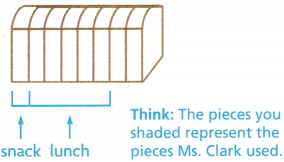So, Ms. Clark used _________ eighth-size
pieces, or $$\frac{}{8}$$ of the bread.
$$\frac{1}{8}$$ is 1 by eighth-size piece of bread.
$$\frac{5}{8}$$ is 5 by  eighth-size pieces of bread.So, Ms. Clark used 6 by eighth-size
pieces, or $$\frac{6}{8}$$ of the bread.

Another Way Use fraction strips.

The 1 strip represents the whole loaf.
Each $$\frac{1}{8}$$ part represents 1 eighth-size piece of bread.
Shade $$\frac{1}{8}$$. Then shade $$\frac{5}{8}$$.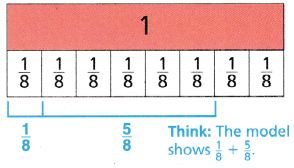How many $$\frac{1}{8}$$-size parts are shaded? _____________
Write the sum. $$\frac{1}{8}+\frac{5}{8}$$ = $$\frac{}{8}$$
So, Ms. Clark __________ used of the bread.
How many $$\frac{1}{8}$$-size parts are shaded? $$\frac{6}{8}$$ parts are shaded
Write the sum. $$\frac{1}{8}+\frac{5}{8}$$ = $$\frac{6}{8}$$
So, Ms. Clark $$\frac{6}{8}$$ used of the bread.

Math Talk

Mathematical Processes
Explain why $$\frac{1}{8}+\frac{5}{8}$$ ≠ $$\frac{6}{16}$$
Answer: Because the denominators are different
Explanation:
As the denominators are different
That means the number of parts divided are different.

Make Connections

Question 1.
Explain how the numerator of the sum is related to the fraction strip model.
Numerator shows How many parts are completed are how many parts to be completed in the fraction strip model
If the denominators are unlike the numerators cannot be added directly

Question 2.
Explain how the denominator of the sum is related to the fraction strip model.
The denominator shows how many parts are there in total
If the denominators are same we can directly add the numerator.

Example
Jacob needs two strips of wood to make masts for a miniature sailboat. One mast will be $$\frac{3}{6}$$ foot long. The other mast will be $$\frac{2}{6}$$ foot long. He has a strip of wood that is $$\frac{4}{6}$$ foot long. Is this strip of wood long enough to make both masts?
Explanation: $$\frac{3}{6}+\frac{2}{6}$$ = $$\frac{5}{6}$$
$$\frac{4}{6}$$ < $$\frac{5}{6}$$
so, strip of wood long not enough to make both masts

Shade the model to show $$\frac{3}{6}+\frac{2}{6}$$Write the sum. $$\frac{3}{6}+\frac{2}{6}$$ = $$\frac{}{6}$$
Is the sum less than or greater than $$\frac{4}{6}$$? _____________
So, the strip of wood ______________ long enough to make both masts.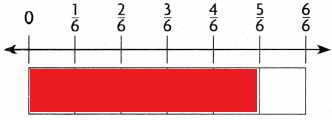$$\frac{3}{6}+\frac{2}{6}$$ = $$\frac{5}{6}$$
Is the sum less than or greater than $$\frac{4}{6}$$? Greater than $$\frac{4}{6}$$
So, the strip of wood not long enough to make both masts.

Question 3.
Explain how you used the number line to determine if the sum was less than $$\frac{4}{6}$$.
Answer: $$\frac{4}{6}$$ < $$\frac{5}{6}$$.
Explanation:
The sum was not less than $$\frac{4}{6}$$.
It is greater than $$\frac{4}{6}$$.

Question 4.
What if each mast was $$\frac{4}{6}$$ foot long? Could Jacob use the strip of wood to make both masts? Explain.
The wood will not be suffitient.

Share and Show

Question 1.
Gary’s cat ate $$\frac{3}{5}$$ of a hag of cat treats in September and $$\frac{1}{5}$$ of the same bag of cat treats in October. What part of the bag of cat treats did Gary’s cat eat in both months?
Use the model to find the sum $$\frac{3}{5}+\frac{1}{5}$$.
How many fifth-size pieces are shown? _________
$$\frac{3}{5}+\frac{1}{5}$$ = $$\frac{}{5}$$ of a bag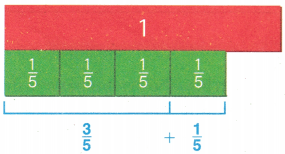$$\frac{3}{5}+\frac{1}{5}$$ = $$\frac{4}{5}$$
Explanation:
With the help of fraction strip model we can see that
$$\frac{3}{5}+\frac{1}{5}$$ = $$\frac{4}{5}$$ part of the bag of cat treats has Gary’s cat eat in both months

Use the model to find the sum.

Question 2.$$\frac{1}{4}+\frac{2}{4}$$ = __________
$$\frac{1}{4}+\frac{2}{4}$$ =  $$\frac{3}{4}$$
Explanation:
With the help of fraction strip model founded the equation.

Question 3.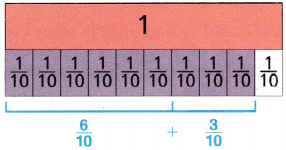$$\frac{6}{10}+\frac{3}{10}$$ = ___________
$$\frac{6}{10}+\frac{3}{10}$$ = $$\frac{9}{10}$$
Explanation:
With the help of fraction strip model founded the equation.

Find the sum. Use models to help.

Question 4.
$$\frac{3}{6}+\frac{3}{6}$$ = ___________
$$\frac{3}{6}+\frac{3}{6}$$ = 1
Explanation:
With the help of fraction strip model founded the equation.

Question 5.
$$\frac{5}{8}+\frac{2}{8}$$ = ___________
$$\frac{5}{8}+\frac{2}{8}$$ = $$\frac{7}{8}$$
Explanation:
With the help of fraction strip model founded the equation.

Question 6.
$$\frac{1}{3}+\frac{1}{3}$$ = ___________
$$\frac{1}{3}+\frac{1}{3}$$ = $$\frac{2}{3}$$
Explanation:
With the help of fraction strip model founded the equation.

Math Talk

Mathematical Processes
ExpIain how to add $$\frac{2}{6}+\frac{3}{6}$$.
$$\frac{2}{6}+\frac{3}{6}$$. = $$\frac{5}{6}$$
Explanation:
As the denominators are same we directly add the numerators.
With the help of fraction strip model founded the equation.

Problem Solving

Question 7.
Write Math
Use Math Language Jin is putting colored sand in a jar. She filled $$\frac{2}{10}$$ of the jar with blue sand and $$\frac{4}{10}$$ of the jar with pink sand. Describe one way to model the part of the jar filled with sand.Explanation:
With the help of fraction strip model we can say that
$$\frac{2}{10}$$ + $$\frac{4}{10}$$ = $$\frac{6}{10}$$

Question 8.
H.O.T. Reasoning A sum has five addends. Each addend is a unit fraction. The sum is 1. What are the addends?
Answer: $$\frac{1}{5}$$
Explanation:
$$\frac{1}{5}$$ are the 5 addends

Question 9.
H.O.T. Multi-Step Mike ate $$\frac{2}{12}$$ of the pizza for dinner. His mom ate $$\frac{1}{12}$$ of the pizza. Mike’s dad ate $$\frac{3}{12}$$ of the pizza. If Mike’s sister ate $$\frac{2}{12}$$ of the pizza, what part of the pizza did Mike and his family eat? Write the answer in simplest form.
$$\frac{2}{12}$$ + $$\frac{1}{12}$$ + $$\frac{3}{12}$$ + $$\frac{2}{12}$$ = $$\frac{8}{12}$$
Explanation:
$$\frac{8}{12}$$ part of the pizza that Mike and his family eat

Question 10.
Schnitzel gets $$\frac{1}{8}$$ cup of dog treats before the race. He gets $$\frac{2}{8}$$ cup of dog treats after the race. How many cups of dog treats does Schnitzel get in all?
(A) $$\frac{3}{8}$$cup
(B) $$\frac{4}{8}$$cup
(C) $$\frac{8}{8}$$cup
(D) $$\frac{2}{8}$$cup
Explanation:
$$\frac{1}{8}$$ + $$\frac{2}{8}$$  = $$\frac{3}{8}$$
$$\frac{3}{8}$$  cups of dog treats that Schnitzel get in all

Question 11.
Kelly has a seashell collection. Of them, $$\frac{5}{12}$$ of the shells are white
and $$\frac{2}{12}$$ of the shells are pink. What fraction of the seashells in Kelly’s collection are white or pink?
(A) $$\frac{6}{12}$$
(B) $$\frac{3}{12}$$
(C) $$\frac{7}{12}$$
(D) $$\frac{12}{12}$$
Explanation:
$$\frac{5}{12}$$ + $$\frac{2}{12}$$ = $$\frac{7}{12}$$
Used the simplest form

Question 12.
Multi-Step Jason is making a fruit drink. He mixes $$\frac{2}{8}$$ quart of grape juice with $$\frac{3}{8}$$ quart of apple juice. Then he adds $$\frac{1}{8}$$ quart of lemonade. How much fruit drink does Jason make?
(A) $$\frac{4}{8}$$quart
(B) $$\frac{5}{8}$$quart
(C) $$\frac{8}{8}$$quart
(D) $$\frac{6}{8}$$quart
Explanation:
$$\frac{2}{8}$$ + $$\frac{3}{8}$$  + $$\frac{1}{8}$$  = $$\frac{6}{8}$$quart
Jason make $$\frac{6}{8}$$quart juice.

TEXAS Test Prep

Question 13.
Jamal is making some dessert. He adds $$\frac{2}{8}$$ cup of milk to the ingredients. He then adds another $$\frac{3}{8}$$ cup of milk after the ingredients are mixed. How much total milk did Jamal use in the recipe? Use fraction strips or a number line.
(A) $$\frac{1}{8}$$
(B) $$\frac{4}{8}$$
(C) $$\frac{5}{8}$$
(D) $$\frac{6}{8}$$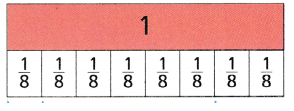Explanation:
$$\frac{2}{8}$$ + $$\frac{3}{8}$$ = $$\frac{5}{8}$$

### Texas Go Math Grade 4 Lesson 5.2 Homework and Practice Answer Key

Use the model to find the sum

Question 1.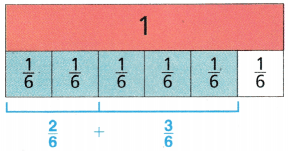$$\frac{2}{6}+\frac{3}{6}$$ = _________
$$\frac{2}{6}+\frac{3}{6}$$ = $$\frac{5}{6}$$
Explanation:
Used the fraction strip model

Question 2.$$\frac{4}{8}+\frac{2}{8}$$ + _________
$$\frac{4}{8}+\frac{2}{8}$$ = $$\frac{6}{8}$$
Explanation:
Used the fraction strip model

Find the sum. Use models to help.

Question 3.
$$\frac{2}{4}+\frac{1}{4}$$ = _________
$$\frac{2}{4}+\frac{1}{4}$$ = $$\frac{3}{4}$$
Explanation:
Used the fraction strip model

Question 4.
$$\frac{5}{7}+\frac{2}{7}$$ = _________
$$\frac{5}{7}+\frac{2}{7}$$ = 1
Explanation:
Used the fraction strip model

Question 5.
$$\frac{1}{5}+\frac{2}{5}$$ = _________
$$\frac{1}{5}+\frac{2}{5}$$  = $$\frac{3}{5}$$
Explanation:
Used the fraction strip model

Question 6.
$$\frac{4}{9}+\frac{3}{9}$$ = _________
$$\frac{4}{9}+\frac{3}{9}$$ = $$\frac{7}{9}$$
Explanation:
Used the fraction strip model

Problem Solving

Question 7.
Leigh is layering cookie ingredients in a jar. She fills $$\frac{3}{8}$$ of the jar with flour arid then $$\frac{1}{8}$$ of the jar with sugar. how much of the jar has Lee filled so far? Draw a model to find the sum.
$$\frac{3}{8}$$ + $$\frac{1}{8}$$ = $$\frac{4}{8}$$Explanation:
$$\frac{4}{8}$$ jar has Lee filled so far

Question 8.
Kevin is adding toppings to a pizza. He adds mushrooms to $$\frac{2}{6}$$ of the pizza and olives to $$\frac{3}{6}$$ of the pizza. What fraction of the pizza has a topping on it? Draw a model to find the sum.$$\frac{2}{6}$$ + $$\frac{3}{6}$$ = $$\frac{5}{6}$$
Explanation:
$$\frac{5}{6}$$ fraction of the pizza has a topping on it

Lesson Check

Question 9.
Jason’s rain gauge showed $$\frac{1}{4}$$ inch of rain on Saturday and $$\frac{3}{4}$$ inch of rain on Sunday. What is the total amount of rainfall received?
(A) $$\frac{3}{4}$$inch
(B) $$\frac{2}{4}$$inch
(C) 1 inch
(D) $$\frac{4}{8}$$
Explanation:
$$\frac{1}{4}$$  + $$\frac{3}{4}$$  = 1
The total amount of rainfall received is 1 inch

Question 10.
Julie read $$\frac{2}{8}$$ of lier book before lunch and $$\frac{5}{8}$$ of her book after lunch. What fraction of the book has Julie read?
(A) $$\frac{3}{8}$$
(B) $$\frac{7}{8}$$
(C) $$\frac{6}{8}$$
(D) $$\frac{8}{8}$$
Explanation:
$$\frac{2}{8}$$ + $$\frac{5}{8}$$  = $$\frac{7}{8}$$
$$\frac{7}{8}$$ fraction of the book has Julie read

Question 11.
Frank displays framed photos on a wall. Of the photos, $$\frac{5}{12}$$ are in wooden frames and $$\frac{2}{12}$$ are in metal frames. What fraction shows the number of photos that are in either wooden or metal frames?
(A) $$\frac{8}{12}$$
(B) $$\frac{3}{12}$$
(C) $$\frac{6}{12}$$
(D) $$\frac{7}{12}$$
Explanation:
$$\frac{5}{12}$$ + $$\frac{2}{12}$$ = $$\frac{7}{12}$$
$$\frac{7}{12}$$ fraction shows the number of photos that are in either wooden or metal frames

Question 12.
Anish painted $$\frac{5}{10}$$ of a fence on Saturday and $$\frac{2}{10}$$ of the fence on Sunday. How much of the fence was painted by the end of the weekend?
(A) $$\frac{7}{10}$$
(B) $$\frac{2}{5}$$
(C) $$\frac{3}{10}$$
(D) $$\frac{4}{5}$$
Explanation:
$$\frac{5}{10}$$  + $$\frac{2}{10}$$  = $$\frac{7}{10}$$
$$\frac{7}{10}$$ of the fence was painted by the end of the weekend

Question 13.
Multi-Step Bonnie spent $$\frac{2}{10}$$ of her money on breakfast and $$\frac{3}{10}$$ of her money on lunch. Then she spends $$\frac{4}{10}$$ of her money on dinner. Which fraction shows the amount of money Bonnie has spent today?
(A) $$\frac{7}{10}$$
(B) $$\frac{8}{10}$$
(C) $$\frac{5}{10}$$
(D) $$\frac{9}{10}$$
Explanation:
$$\frac{2}{10}$$ +  $$\frac{3}{10}$$ + $$\frac{4}{10}$$ = $$\frac{9}{10}$$
$$\frac{9}{10}$$ fraction shows the amount of money Bonnie has spent today

Question 14.
Multi-Step After one week, $$\frac{2}{6}$$ of Claire’s flowers bloomed. The second week $$\frac{1}{6}$$ of the flowers bloomed. The third week $$\frac{2}{6}$$ of the flowers bloomed. Which fraction shows the number of flowers that bloomed?
(A) $$\frac{5}{6}$$
(B) $$\frac{4}{6}$$
(C) $$\frac{3}{6}$$
(D) $$\frac{6}{6}$$
$$\frac{2}{6}$$ + $$\frac{1}{6}$$ + $$\frac{2}{6}$$  = $$\frac{5}{6}$$
$$\frac{5}{6}$$ fraction shows the number of flowers that bloomed.